# Concentric Circles

Concentric Circles
Go back to  'Circles'

In this mini-lesson, we will explore the world of concentric circles. We will try answering questions like what are concentric circles, how to calculate area of annulus and discover other interesting aspects of it. You can check out the interactive simulations to know more about the lesson and try your hand at solving a few interesting practice questions at the end of the page.

Now, meet Jennifer. She is a brilliant archer.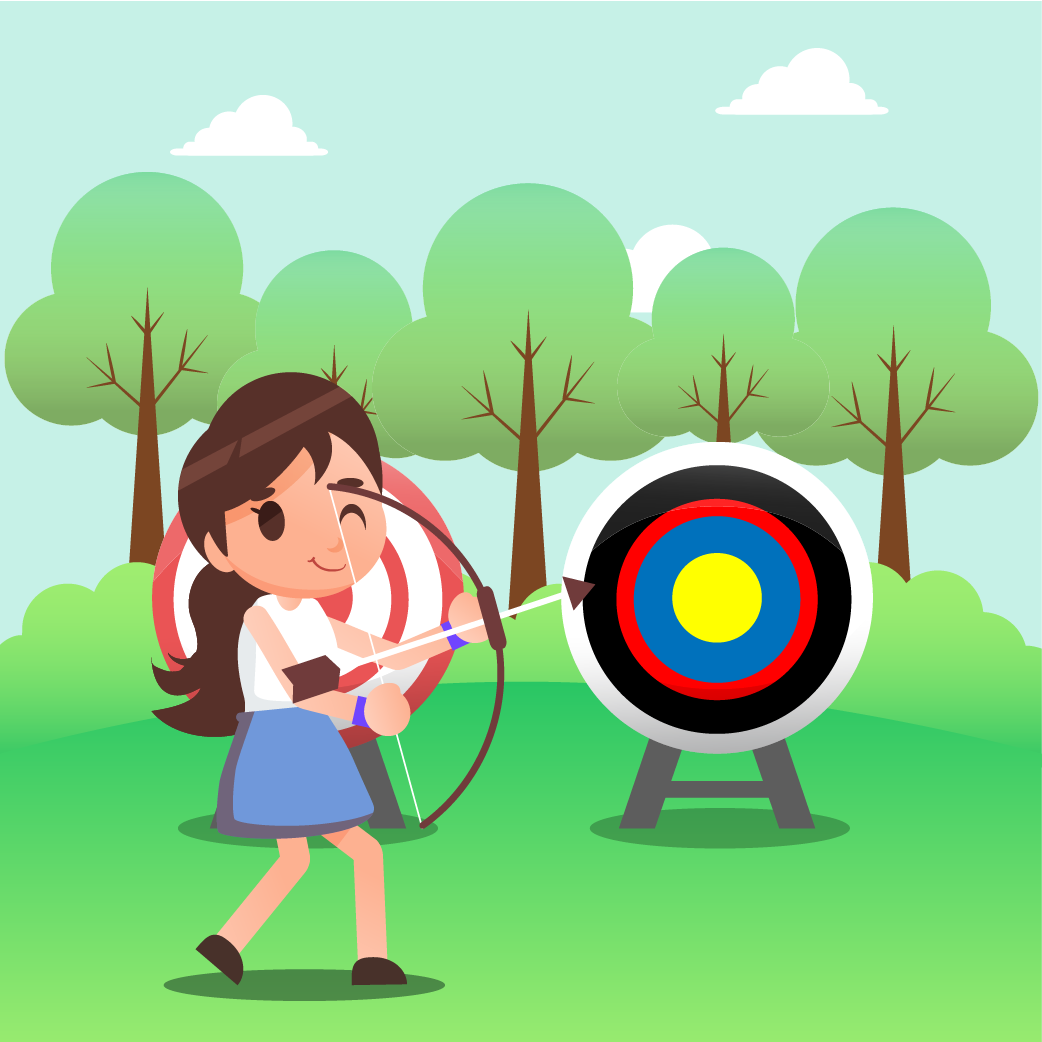You can see that her archery target has 5 scoring regions: Yellow, Blue, Red, Black, and White.

Since you know how to find the area of a circle, you can easily calculate the area of the yellow region.

But can you find the area of all the other bands?

We don't want you to worry, as we, at Cuemath, understand how this topic can be confusing for you.

We are here to help you understand this concept in a clear way.

## What Are Concentric Circles?

### Concentric Circles: Definition

Concentric circles are circles with the same center.

The following figure shows two concentric circles with the same center $$O$$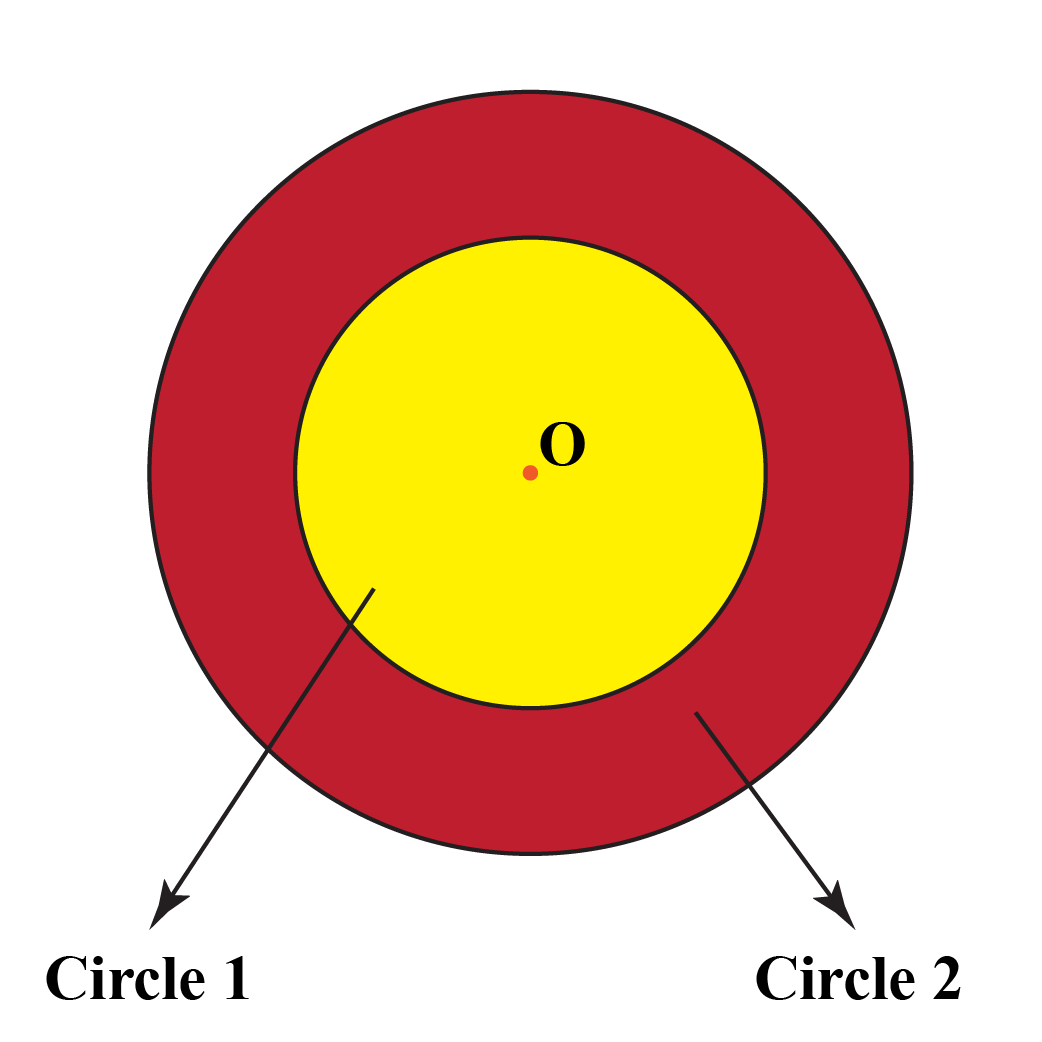Experiment with the simulation below to draw concentric circles.

Use the slider to change the radius of the circles with the matching colors.

So, which color gives you the largest radius?

## Area of Annulus: The Region Between Concentric Circles

An area/region formed between two concentric circles is called an annulus.

It is flat shaped, like a ring.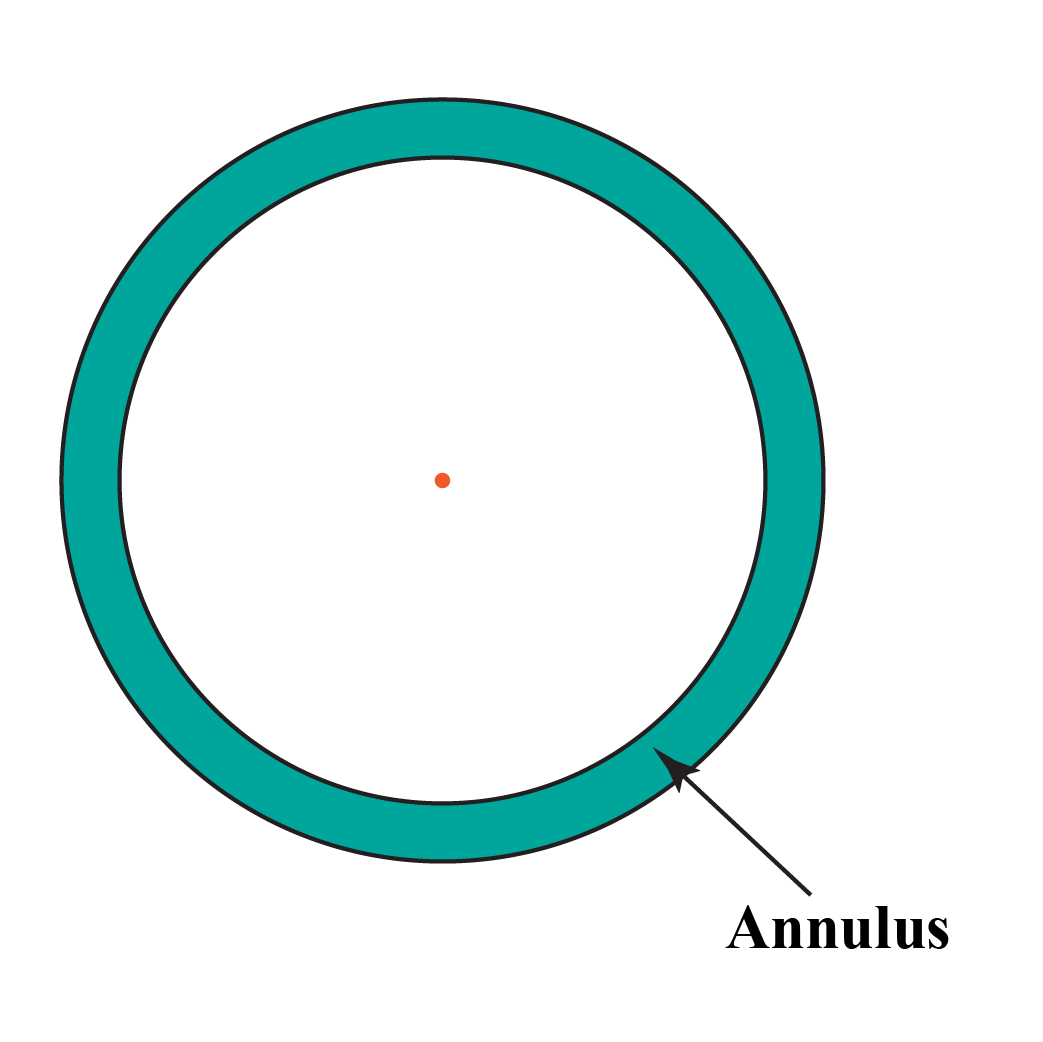Look at the following figure.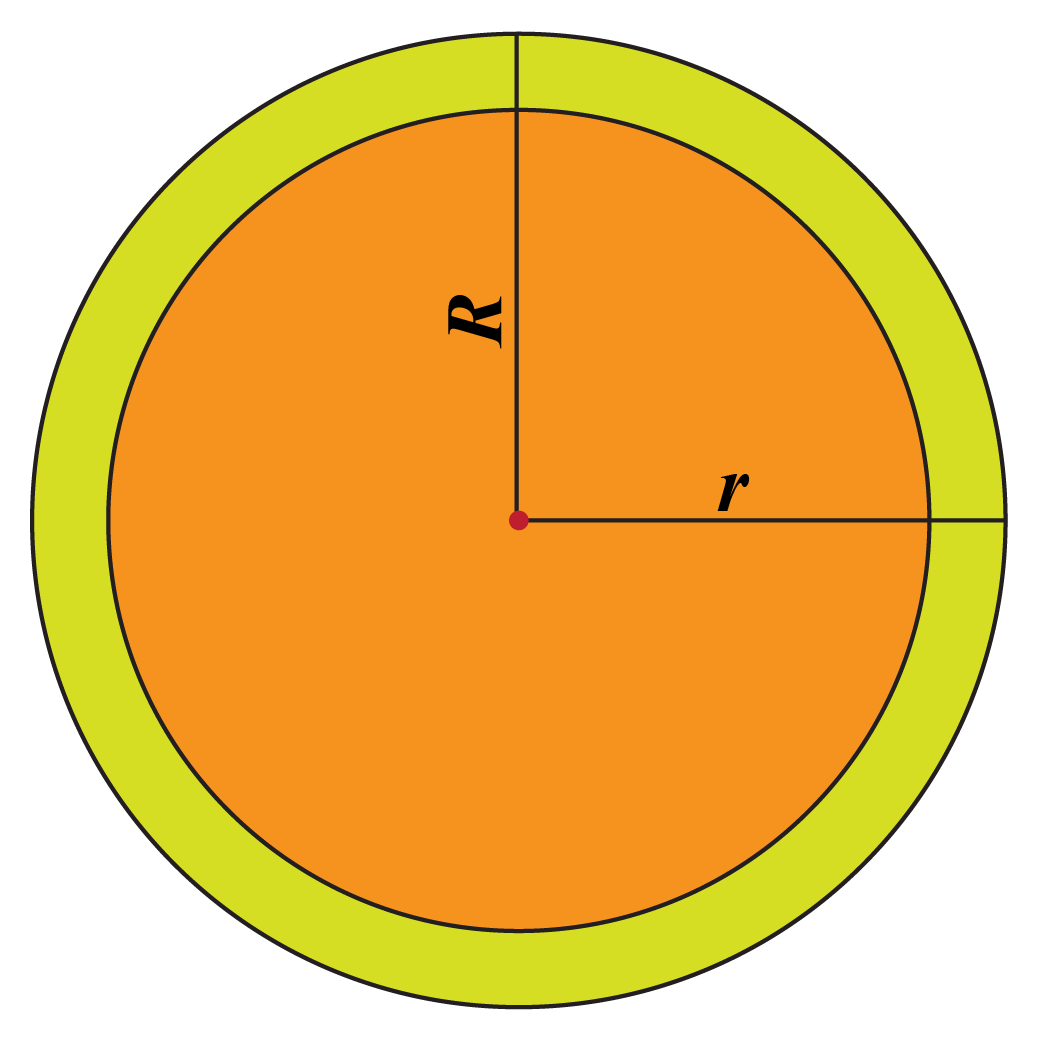Clearly, we can find the area of the red region by subtracting the area of a small circle from that of the large circle.

The radius of the small circle is $$r$$ and the radius of the large circle is $$R$$.

So, the area of the annulus (red region) can be calculated using the formula:

 $\pi R^{2}-\pi r^{2}=\pi (R^{2}-r^{2})$

## Solved Example on Area Of Annulus

 Example 1

Jimmy runs on a path that is 14 inches wide.

The path is surrounded by a circular lawn of diameter 360 inches.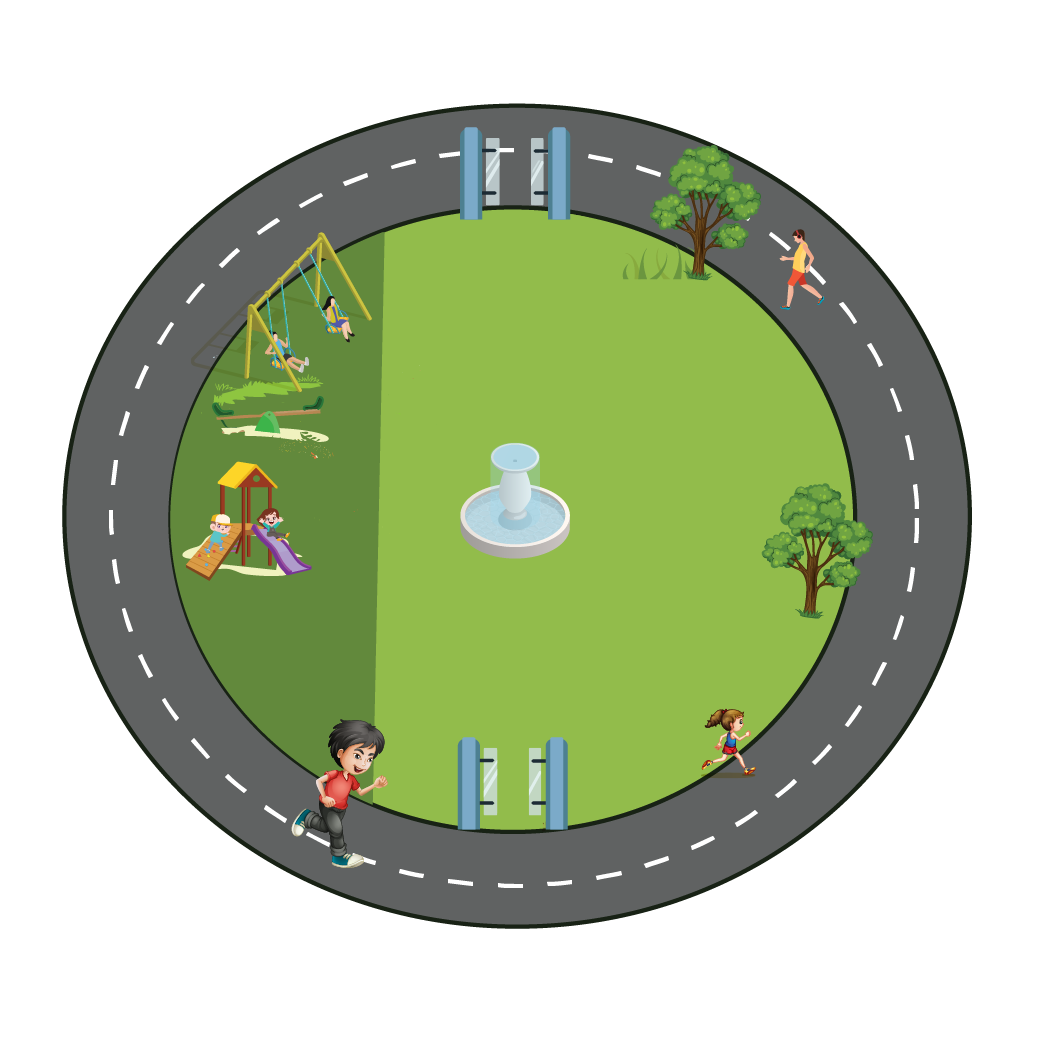Can you determine the area of the path?

Solution

The radius of inner circle is $$r=\dfrac{360}{2}\;\text{inches}=180\;\text{inches}$$.

The radius of outer circle is $$R=180\;\text{inches}+14\;\text{inches}=194\;\text{inches}$$.

The area of the path will be given by the formula $$\pi (R^{2}-r^{2})$$.

\begin{align}\text{Area of path}&=\pi (R^{2}-r^{2})\\&=\dfrac{22}{7} (194^{2}-180^{2})\\&=\dfrac{22}{7} \times 5236\\&=16456\end{align}

 $$\therefore$$ The area of the path is $$16456\;\text{inches}^2$$.
 Example 2

A race-track is in the form of a circular ring. The inner radius of the track is 58 inches and the outer radius is 63 inches.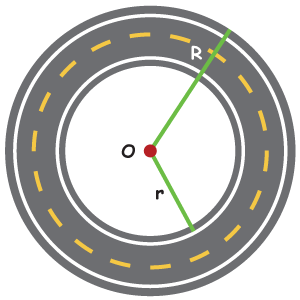Find the area of the race-track.

Solution

Given that, $$R$$ = 63 inches and $$r$$ = 56 inches

Let the area of the outer circle be $$A_1$$ and the area of the inner circle be $$A_2$$.

The area of race track is calculated as:

\begin{align} &= (\text A_1) - (\text A_2) \\ & = \pi(R)^2 - \pi (r)^2 \\ & = \pi (63^2 -56^2) \\ & = \left(\frac{22}{7}\right) \times 833 \\ & = 2618 \text {inches}^2 \end{align}

 $$\therefore$$ Area of the Race-track $$= 2618 \text { inches}^2$$

## Concentric Circle Examples In Real Life

Have you ever observed wheel of a ship?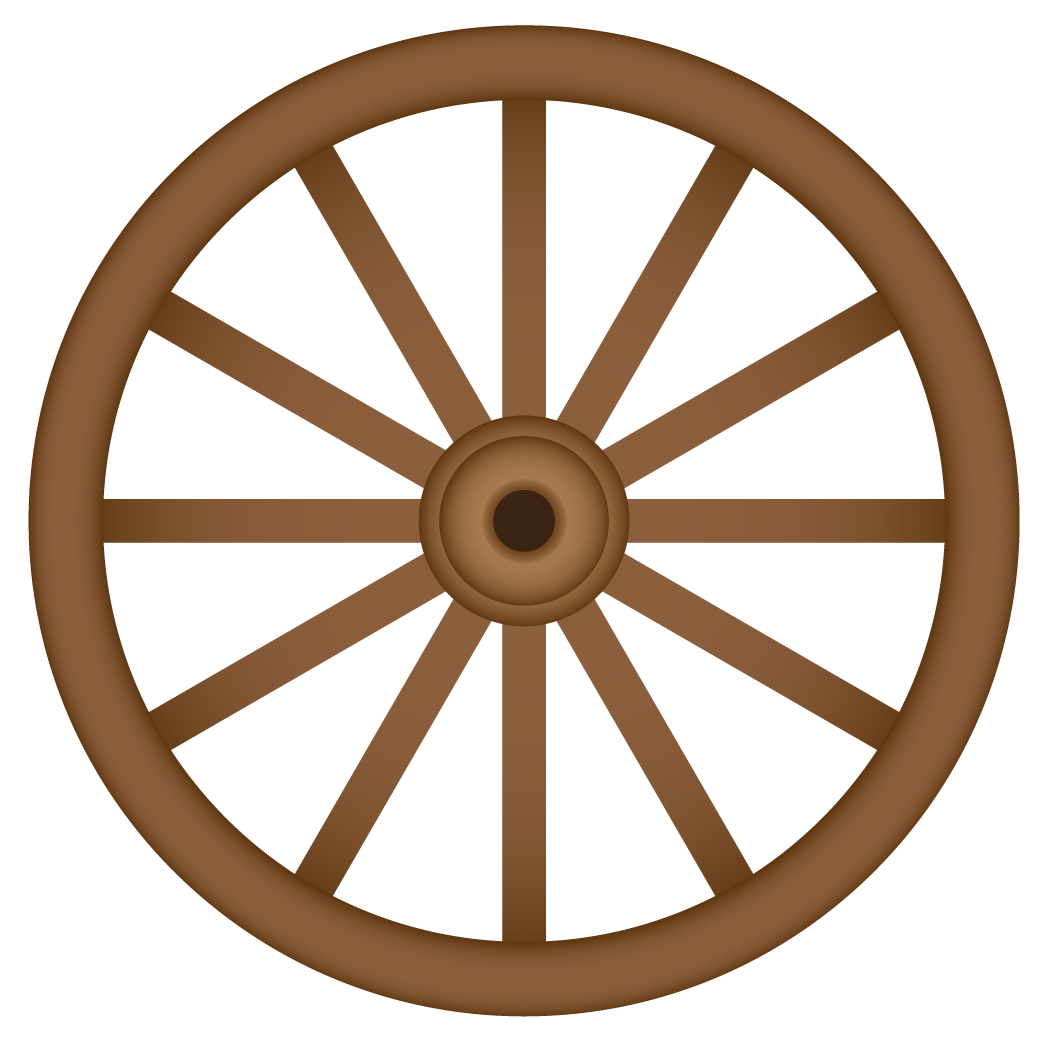It has two concentric circles joined by the spokes.

Many of us have played darts. A dartboard is an example of concentric circles.

Observe that the circles in the dartboard are drawn around a common center, that is, bullseye.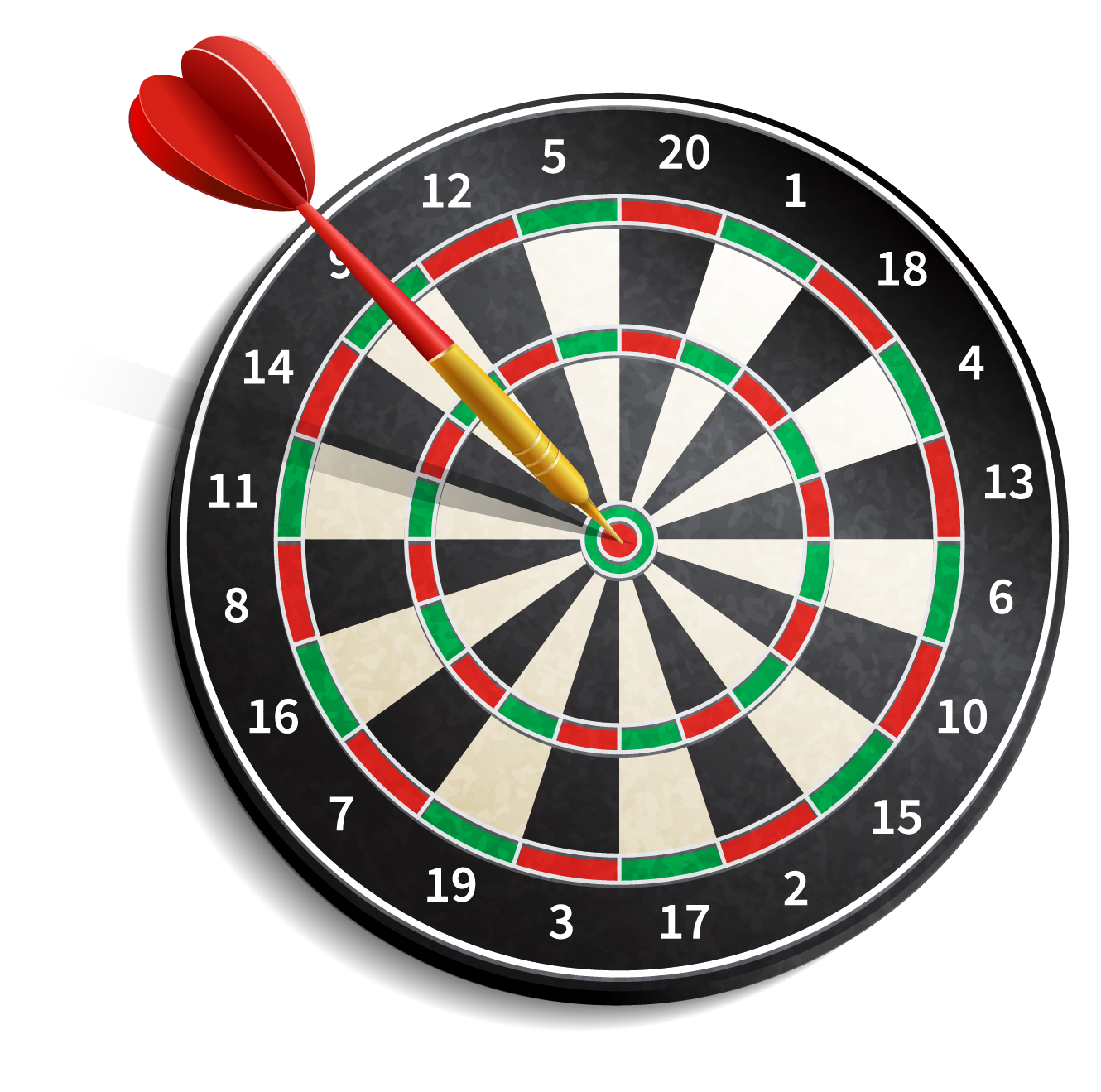Now, look at the LP.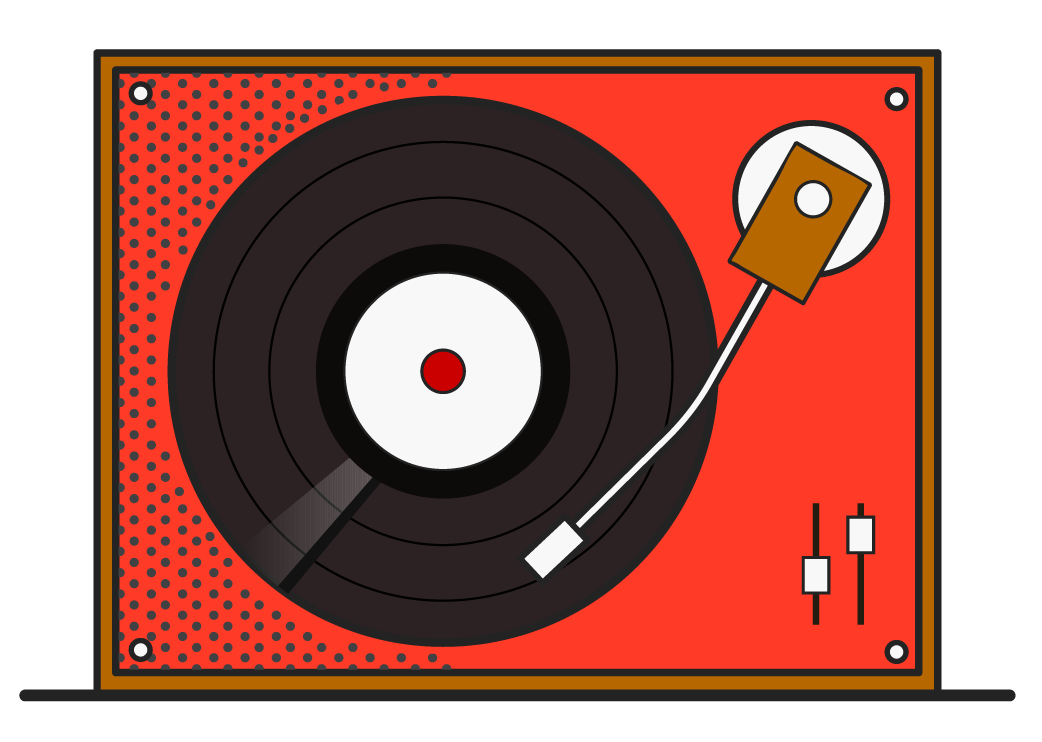The groves that you see on the vinyl record form the concentric circles.Challenging Questions
 1. The radius of the circles is given as 1, 2, 3, 4, and 5 inches. Find the area of the shaded region.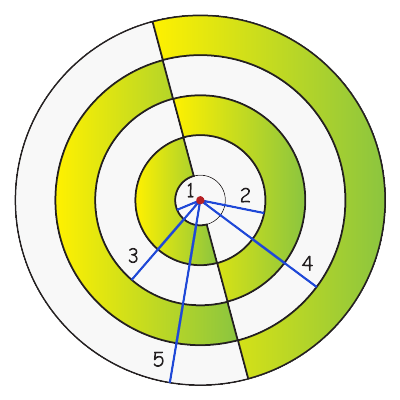## Interactive Questions

Here are a few activities for you to practice.

Select/type your answer and click the "Check Answer" button to see the result.

## Let's Summarize

We hope you enjoyed learning about concentric circles with the examples and practice questions. Now, you will be able to easily solve problems on the concentric circles.

## About Cuemath

At Cuemath, our team of math experts is dedicated to making learning fun for our favorite readers, the students!

Through an interactive and engaging learning-teaching-learning approach, the teachers explore all angles of a topic.

Be it worksheets, online classes, doubt sessions, or any other form of relation, it’s the logical thinking and smart learning approach that we, at Cuemath, believe in.

## 1. What is the area between concentric circles?

The area between two concentric circles is annulus.

## 2. What is Annulus?

The area bounded by two concentric circles is called an annulus.

## 3. How many points are shared by concentric circles?

Concentric circles share no common points.

More Important Topics
Numbers
Algebra
Geometry
Measurement
Money
Data
Trigonometry
Calculus